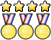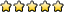## Geologic Mapping C

syo_astro
Exalted MemberPosts: 601
Joined: December 3rd, 2011, 9:45 pm
State: NY
Has thanked: 0
Been thanked: 5 times
Contact:

### Re: Geologic Mapping C

Unome wrote:
Qu€€nMon€y wrote:The rule says tracing paper and pin. What kind of pin?
The ideal pin would of the capped type sometimes used with nametags. Any type of pin with a flat head should work, provided you can avoid being poked by the tip pointing upwards.
I believe I mentioned an example on the first page: viewtopic.php?f=285&t=12238#p359151 as well as noting that you should find example videos on youtube to see the process (which should help show you why / the type of pin you need): viewtopic.php?f=285&t=12238#p359155. Note for both those cases those are just examples, not necessarily the best or cheapest (aka I was just putting that together quickly as examples, you should look into it yourself of course).

Not sure what Unome means by capped pin for nametags (maybe/probably same thing?), but hopefully that should work/helps. Specify if it doesn't, etc!
B: Crave the Wave, Environmental Chemistry, Robo-Cross, Meteorology, Physical Science Lab, Solar System, DyPlan (E and V), Shock Value
C: Microbe Mission, DyPlan (Earth's Fresh Waters), Fermi Questions, GeoMaps, Gravity Vehicle, Scrambler, Rocks, Astronomy

poonicle
MemberPosts: 23
Joined: September 22nd, 2017, 3:11 pm
Division: C
Has thanked: 0
Been thanked: 0

### Re: Geologic Mapping C

On one test, the formula for true thickness was given as T = Wsin(d) + Hcos(d) where T = true thickness, W = width, H = height, d = dip, whereas on another test, the formula for true thickness was given as T = Wsin(d) where T = true thickness, W = width, d = dip. Which one is the correct formula? I am very confused.
Events: WIDI, Geomap, Disease, Chem Lab
Acton-Boxborough A for Invites season

UTF-8 U+6211 U+662F
Exalted MemberPosts: 1523
Joined: January 18th, 2015, 7:42 am
Division: C
State: PA
Has thanked: 1 time
Been thanked: 1 time

### Re: Geologic Mapping C

poonicle wrote:On one test, the formula for true thickness was given as T = Wsin(d) + Hcos(d) where T = true thickness, W = width, H = height, d = dip, whereas on another test, the formula for true thickness was given as T = Wsin(d) where T = true thickness, W = width, d = dip. Which one is the correct formula? I am very confused.
What is height?

(Note that a formula for true thickness can be derived using basic trig in emergencies!)

Unome
ModeratorPosts: 4253
Joined: January 26th, 2014, 12:48 pm
State: GA
Location: somewhere in the sciolyverse
Has thanked: 85 times
Been thanked: 32 times

### Re: Geologic Mapping C

UTF-8 U+6211 U+662F wrote:
poonicle wrote:On one test, the formula for true thickness was given as T = Wsin(d) + Hcos(d) where T = true thickness, W = width, H = height, d = dip, whereas on another test, the formula for true thickness was given as T = Wsin(d) where T = true thickness, W = width, d = dip. Which one is the correct formula? I am very confused.
What is height?

(Note that a formula for true thickness can be derived using basic trig in emergencies!)
I would strongly recommend deriving the formula yourself, so you understand how it works. There are several standard formulas for true thickness that cover various situations.
Userpage
Chattahoochee High School Class of 2018
Georgia Tech Class of 2022

Opinions expressed on this site are not official; the only place for official rules changes and FAQs is soinc.org.

Snayhill
MemberPosts: 5
Joined: July 6th, 2018, 9:58 am
Division: C
State: OH
Has thanked: 0
Been thanked: 0

### Re: Geologic Mapping C

Hello Everyone!

I was just wondering if anyone here know what lines that are not faults in a relative dating/strata diagram are. Here's a link https://d2vlcm61l7u1fs.cloudfront.net/m ... IiQrsg.png. I'm specifically talking about "G" in this picture.

Thanks!

UTF-8 U+6211 U+662F
Exalted MemberPosts: 1523
Joined: January 18th, 2015, 7:42 am
Division: C
State: PA
Has thanked: 1 time
Been thanked: 1 time

### Re: Geologic Mapping C

Snayhill wrote:Hello Everyone!

I was just wondering if anyone here know what lines that are not faults in a relative dating/strata diagram are. Here's a link https://d2vlcm61l7u1fs.cloudfront.net/m ... IiQrsg.png. I'm specifically talking about "G" in this picture.

Thanks!
What does line G represent? It's not a fault because there isn't any relative motion across it as you can see in line K

fabishkf
MemberPosts: 13
Joined: February 17th, 2018, 10:41 am
Division: C
State: WI
Has thanked: 2 times
Been thanked: 0

### Re: Geologic Mapping C

joint
Geology events (RIP Rocks and Minerals 2021)

bluesm0ke
MemberPosts: 1
Joined: January 21st, 2019, 8:07 pm
Has thanked: 0
Been thanked: 0

### Re: Geologic Mapping C

Unome wrote:
UTF-8 U+6211 U+662F wrote:
poonicle wrote:On one test, the formula for true thickness was given as T = Wsin(d) + Hcos(d) where T = true thickness, W = width, H = height, d = dip, whereas on another test, the formula for true thickness was given as T = Wsin(d) where T = true thickness, W = width, d = dip. Which one is the correct formula? I am very confused.
What is height?

(Note that a formula for true thickness can be derived using basic trig in emergencies!)
I would strongly recommend deriving the formula yourself, so you understand how it works. There are several standard formulas for true thickness that cover various situations.
Do you think you could post those formulas here? I'm having a hard time grappling more complex true thickness problems that don't just use the basic formula. thanks

Unome
ModeratorPosts: 4253
Joined: January 26th, 2014, 12:48 pm
State: GA
Location: somewhere in the sciolyverse
Has thanked: 85 times
Been thanked: 32 times

### Re: Geologic Mapping C

bluesm0ke wrote:
Unome wrote:
UTF-8 U+6211 U+662F wrote: What is height?

(Note that a formula for true thickness can be derived using basic trig in emergencies!)
I would strongly recommend deriving the formula yourself, so you understand how it works. There are several standard formulas for true thickness that cover various situations.
Do you think you could post those formulas here? I'm having a hard time grappling more complex true thickness problems that don't just use the basic formula. thanks
I don't have the third one memorized, and can't seem to find it, but I'm pretty sure it's on one of the test exchange tests.
Userpage
Chattahoochee High School Class of 2018
Georgia Tech Class of 2022

Opinions expressed on this site are not official; the only place for official rules changes and FAQs is soinc.org.

poonicle
MemberPosts: 23
Joined: September 22nd, 2017, 3:11 pm
Division: C
Has thanked: 0
Been thanked: 0

### Re: Geologic Mapping C

bluesm0ke wrote:
Unome wrote:
UTF-8 U+6211 U+662F wrote: What is height?

(Note that a formula for true thickness can be derived using basic trig in emergencies!)
I would strongly recommend deriving the formula yourself, so you understand how it works. There are several standard formulas for true thickness that cover various situations.
Do you think you could post those formulas here? I'm having a hard time grappling more complex true thickness problems that don't just use the basic formula. thanks
yeah, i'm not gonna lie, i asked that question before TSTs when i didn't know that much about the event. my accuracy improved a lot when i started drawing stuff out then applying trig as opposed to formulas--i'm not very good at identifying which formula applies to which situation yet, but drawing a picture really, really helps imo

that being said, i found T = w*sin(D - S) where D = dip, S = slope, T = true thickness on one practice test. hope that's helpful
Events: WIDI, Geomap, Disease, Chem Lab
Acton-Boxborough A for Invites season

### Who is online

Users browsing this forum: No registered users and 1 guest